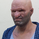552 views
552
Normalization often involve the ratio of a quantity over a variable in order to bring that quantity in a specific scale (fixed/non-fixed). Normalized oscillators are common types of oscillators used in technical analysis .

The proposed indicator is a normalized oscillator in a scale (-1,1) and is based on the average error between the price and a specific moving average divided by the average absolute error. The indicator can be smoother by checking the "smoother" option.

The interpretation is the same as any other type of oscillators, overbought/sold levels are also provided (-0.8,0.8) by default.hi alex i'm trying to use you quadratic regression ma instead of a sma in your average absolute error normalization, but it is not acting as i expected. i have used a zlema and it works great. i am just trying to take a little more lag out of it. any suggestions?

``` //@version=4
study("Average Absolute Error Normalization","AEN")
length = input(18, step = 1)
tol = input(0.8, title = "Tolerance", step = 0.01)
smo = input(false,"Smoother")
src = close
//----

x1 = bar_index
x2 = pow(x1, 2)
S11 = sum(x2, length) - pow(sum(x1, length), 2) / length
S12 = sum(x1 * x2, length) - sum(x1, length) * sum(x2, length) / length
S22 = sum(pow(x2, 2), length) - pow(sum(x2, length), 2) / length
Sy1 = sum(y * x1, length) - sum(y, length) * sum(x1, length) / length
Sy2 = sum(y * x2, length) - sum(y, length) * sum(x2, length) / length
max1 = sma(x1, length)
max2 = sma(x2, length)
may = sma(y, length)
b2 = (Sy1 * S22 - Sy2 * S12) / (S22 * S11 - pow(S12, 2))
b3 = (Sy2 * S11 - Sy1 * S12) / (S22 * S11 - pow(S12, 2))
b1 = may - b2 * max1 - b3 * max2
Y = b1 + b2 * x1 + b3 * x2

//----
y = 0.
y := quadReg(src + iff(smo,a*e,0),length) + iff(smo,0,a*e)
//----
//col = rising(a,1)? color.blue : falling(a,1) ? color.red : color.white
col = a >= tol ? color.red : a <= tol*-1 ? color.green : color.yellow
bgcolor(a > 0 ? color.new(color.green,80): a < 0 ? color.new(color.red,80) : color.yellow)

plot(a,"Plot",color=col, transp=20, linewidth = 2)
hline(tol,color = color.new(color.orange,50),linewidth=1, linestyle = hline.style_dotted)
hline(tol*-1,color= color.new(color.orange,50),linewidth=1, linestyle = hline.style_dotted)
hline(0,color= color.new(color.orange,50),linewidth=1, linestyle = hline.style_dotted)
```